# Model verification

## Introduction

This document aims at verifying the implementation of the Lemna toxicokinetic-toxicodynamic (TKTD) model as described by Klein et al. (2022). The model is a refined description of the Lemna TKTD model based on Schmitt et al. (2013).

First, this model’s output is compared to published results which were created with the reference implementation of the Schmitt et al. model. For unlimited growth scenarios, both models are expected to yield identical results for identical inputs. Second, model dynamics are compared for additional scenarios which simulate changing environmental conditions, such as under field study conditions. In this case, the models are not expected to yield identical but equivalent behavior due to differences between the models’ response functions.

## Schmitt et al. model

The outputs of the Lemna TKTD model by Schmitt et al. are used to verify the implementation of the Klein et al. model. The reference implementation of Schmitt et al. is included in this package’s GitHub repository but is not an official part of the package.

Sourcing files mmc2.r and mmc3.r gives access to the Schmitt et al. model equations as well as to the fitted parameter set for the substance metsulfuron-methyl.

library(lemna)   # lemna model
# load packages to ease plotting and data wrangling
library(dplyr)   # for data preparation
library(ggplot2) # for plotting

# load model files by Schmitt et al. (2013)
source("mmc2.r")
source("mmc3.r")

# run sample simulation with metsulfuron-methyl parameters
calcgrowth(timepoints = 0:7,
vars = c(BM = 0.0012, E=1, M_int=0),
param = param, # default scenario data
)
#>   time          BM       M_int     C_int  FrondNo
#> 1    0 0.001200000 0.000000000 0.0000000 12.00000
#> 2    1 0.001284774 0.005436458 0.2533803 12.84774
#> 3    2 0.001306316 0.009105453 0.4173850 13.06316
#> 4    3 0.001314398 0.011494598 0.5236614 13.14398
#> 5    4 0.001320074 0.013044177 0.5917003 13.20074
#> 6    5 0.001324988 0.014053436 0.6351173 13.24988
#> 7    6 0.001329581 0.014716554 0.6627886 13.29581
#> 8    7 0.001334016 0.015158319 0.6804143 13.34016

## Unlimited growth scenarios

This section simulates several Lemna growth scenarios under unlimited growth conditions using the Klein et al. model. Model dynamics and numerical results are compared to results of the Schmitt et al. model.

### Seven days exposure, seven days recovery

Figure 2 in Schmitt et al. (2013) presents several scenarios which simulate the growth of Lemna exposed to several concentrations of metsulfuron-methyl. Exposure lasts for seven days, followed by a seven day recovery period. Symbols show observed data and lines as calculated with fitted model parameters.

# simulate the 7d exposure, 7d recovery experiment
simulate_7d <- function(model=c("schmitt","klein"), ...) {
model <- match.arg(model)
time <- seq(0, 14, 0.2) # output time points
levels <- c(0, 0.32, 0.56, 1, 1.8, 3.2, 5.6) # simulated exposure levels

# set parameters as needed to replicate Figure 2 from Schmitt et al.
if(model == "schmitt") {
param$k_phot_fix <- TRUE # unlimited growth param$k_phot_max <- 0.4
param$k_resp <- 0 } else if(model == "klein") { param <- metsulfuron$param
param$k_photo_fixed <- TRUE param$k_photo_max <- 0.4
param$k_loss <- 0 envir <- metsulfuron$envir
}

result <- data.frame()
for(conc in levels) {
exposure <- data.frame(time=c(0, 7, 7.01, 14), c=c(conc, conc, 0, 0))

if(model == "schmitt") {
param$Conc <- exposure out <- calcgrowth(time, c(BM=0.0012, E=1, M_int=0), param=param, ...) } else if(model == "klein") { envir$conc <- exposure
out <- lemna(times=time, init=c(BM=0.0012, M_int=0), param=param, envir=envir, ...)
}

out$level = as.character(conc) result <- bind_rows(result, out) } result } # simulate using the Schmitt et al. model df_s <- simulate_7d(model="schmitt") # recreate figure 2 from paper, including observed data ggplot(df_s)+ geom_line(aes(time,FrondNo,color=level))+ geom_point(aes(time,FrondNo,color=level,shape=level), data=schmitt77)+ scale_y_log10(breaks=10^seq(-1,6))+ scale_x_continuous(breaks=seq(0,14,2))+ scale_shape_manual(values=c(0,4,2,8,1,3,5), name="Conc. (ug/L)")+ coord_cartesian(ylim=c(10,10000))+ geom_vline(xintercept=7, linetype="dotted", color="#888888", size=1)+ geom_segment(aes(x=0,y=10000,xend=6.9,yend=10000),arrow=arrow(length=unit(0.3,"cm"),type="closed"),linejoin="mitre",size=1,color="#888888")+ geom_segment(aes(x=7.1,y=10000,xend=14,yend=10000),arrow=arrow(length=unit(0.3,"cm"),type="closed"),linejoin="mitre",size=1,color="#888888")+ geom_text(aes(x=3.5,y=8000,label="Exposure",hjust="middle"),color="#888888")+ geom_text(aes(x=10.5,y=8000,label="Recovery",hjust="middle"),color="#888888")+ theme_bw()+ labs(x="Time (days)", y="Number of Fronds", color="Conc. (ug/L)", title="Schmitt et al. model: 7d exposure, 7d recovery")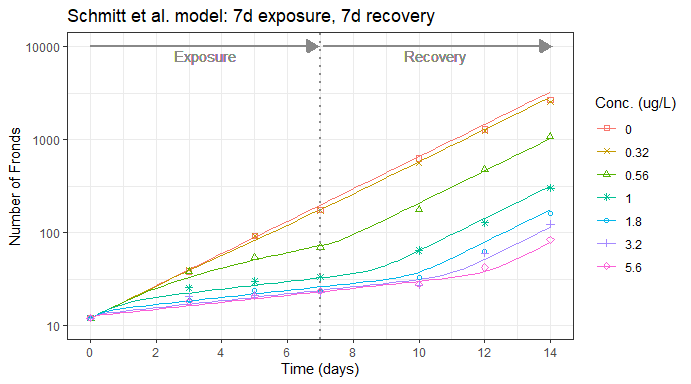The depicted model dynamics of the Schmitt et al. are identical to published results. Simulating the same scenarios using the Klein et al. model yields identical dynamics: # simulate using the Klein et al. model df_k <- simulate_7d(model="klein") # recreate figure 2 from paper, including observed data ggplot(df_k)+ geom_line(aes(time,FrondNo,color=level))+ geom_point(aes(time,FrondNo,color=level,shape=level), data=schmitt77)+ scale_y_log10(breaks=10^seq(-1,6))+ scale_x_continuous(breaks=seq(0,14,2))+ scale_shape_manual(values=c(0,4,2,8,1,3,5), name="Conc. (ug/L)")+ coord_cartesian(ylim=c(10,10000))+ geom_vline(xintercept=7, linetype="dotted", color="#888888", size=1)+ geom_segment(aes(x=0,y=10000,xend=6.9,yend=10000),arrow=arrow(length=unit(0.3,"cm"),type="closed"),linejoin="mitre",size=1,color="#888888")+ geom_segment(aes(x=7.1,y=10000,xend=14,yend=10000),arrow=arrow(length=unit(0.3,"cm"),type="closed"),linejoin="mitre",size=1,color="#888888")+ geom_text(aes(x=3.5,y=8000,label="Exposure",hjust="middle"),color="#888888")+ geom_text(aes(x=10.5,y=8000,label="Recovery",hjust="middle"),color="#888888")+ theme_bw()+ labs(x="Time (days)", y="Number of Fronds", color="Conc. (ug/L)", title="Klein et al. model: 7d exposure, 7d recovery")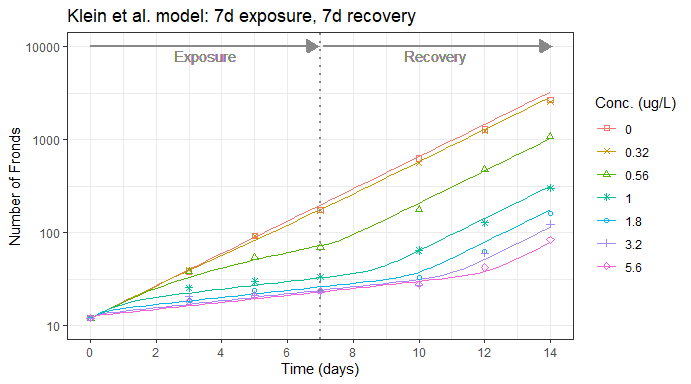A comparison of numerical values between the two models shows that simulated biomass deviates at most by 0.15%. A plot of relative errors describes small but consistent deviations in biomass results: df_s %>% mutate(error = 100 * (df_k$BM/BM - 1)) %>%
ggplot(aes(time, error, color=level)) +
geom_point() +
theme_bw() +
labs(x="Time (days)", y="Relative error (%)", color="Conc. (ug/L)",
title="Deviation in biomass in Klein model relative to Schmitt")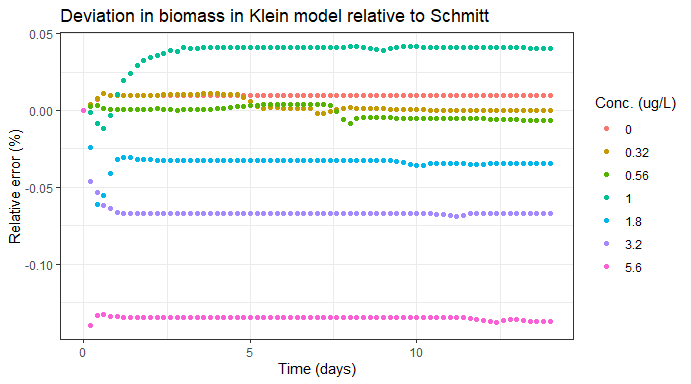The deviations are a result of imprecisions introduced by the numerical ODE solver. Numerical precision can be increased by, for example, decreasing the solver’s step length in time. This can be achieved by decreasing the solver parameter hmax from the model’s default value of hmax=0.1 to e.g. hmax=0.01. The value of 0.01 is equivalent to a step length of about 15 minutes. This decreases the observed deviations significantly to a maximum of about 0.007%:

df_s <-  simulate_7d(model="schmitt", hmax=0.01)
df_k <-  simulate_7d(model="klein", hmax=0.01)

df_s %>%
mutate(error = 100 * (df_k$BM/BM - 1)) %>% ggplot(aes(time, error, color=level)) + geom_point() + theme_bw() + labs(x="Time (days)", y="Relative error (%)", color="Conc. (ug/L)", title="Deviation in biomass in Klein model relative to Schmitt, hmax=0.01")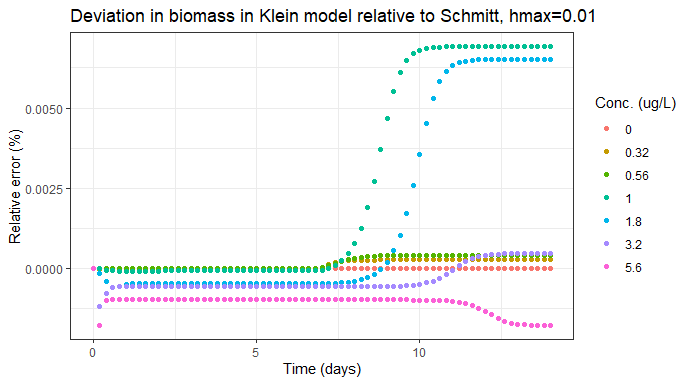It can be concluded that model dynamics and numerical results are identical as far as numerical precision allows. ### Two days of exposure, twelve days of recovery The right-hand side of Figure 3 in Hommen et al. (2015) presents additional scenarios which simulate the growth of Lemna exposed to several concentrations of metsulfuron-methyl. Exposure lasts for two days, followed by a twelve day recovery period. Symbols show observed data and lines as calculated with fitted model parameters: # simulate the 2d exposure, 12d recovery experiment simulate_2d <- function(model=c("schmitt","klein"), ...) { model <- match.arg(model) time <- seq(0, 14, 0.2) # output time points levels <- c(0, 0.35, 0.875, 1.75, 3.5) # simulated exposure levels # set parameters as needed to replicate Figure 3 from Hommen et al. if(model == "schmitt") { param$k_phot_fix <- TRUE # unlimited growth
param$k_phot_max <- 0.295 param$k_resp <- 0.00
param$mass_per_frond <- 0.0004 } else if(model == "klein") { param <- metsulfuron$param
param$k_photo_fixed <- TRUE param$k_photo_max <- 0.295
param$k_loss <- 0 param$r_DW_FN <- 0.0004
envir <- metsulfuron$envir } result <- data.frame() for(conc in levels) { exposure <- data.frame(time=c(0, 2, 2.01, 14), c=c(conc, conc, 0, 0)) if(model == "schmitt") { param$Conc <- exposure
out <- calcgrowth(time, c(BM=12 * param$mass_per_frond, E=1, M_int=0), param=param, ...) } else if(model == "klein") { envir$conc <- exposure
out <- lemna(times=time, init=c(BM=12 * param$r_DW_FN, M_int=0), param=param, envir=envir, ...) } out$level = as.character(conc)
result <- bind_rows(result, out)
}
result
}

# simulate using the Klein et al. model
df_k <- simulate_2d(model="klein")

# recreate figure 3 from paper, including observed data
ggplot(df_k)+
geom_line(aes(time,FrondNo,color=level))+
geom_point(aes(time,FrondNo,color=level,shape=level), data=hommen212)+
scale_y_log10(breaks=10^seq(-1,6))+
scale_x_continuous(breaks=seq(0,14,2))+
scale_shape_manual(values=c(0,4,2,8,1,3,5), name="Conc. (ug/L)")+
coord_cartesian(ylim=c(10,1000))+
geom_vline(xintercept=2, linetype="dotted", color="#888888", size=1)+
geom_segment(aes(x=0,y=1000,xend=1.9,yend=1000),arrow=arrow(length=unit(0.3,"cm"),type="closed"),linejoin="mitre",size=1,color="#888888")+
geom_segment(aes(x=2.1,y=1000,xend=14,yend=1000),arrow=arrow(length=unit(0.3,"cm"),type="closed"),linejoin="mitre",size=1,color="#888888")+
geom_text(aes(x=1,y=800,label="Exposure",hjust="middle"),color="#888888")+
geom_text(aes(x=8,y=800,label="Recovery",hjust="middle"),color="#888888")+
theme_bw()+
labs(x="Time (days)", y="Number of Fronds", color="Conc. (ug/L)",
title="Klein et al. model: 2d exposure, 12d recovery")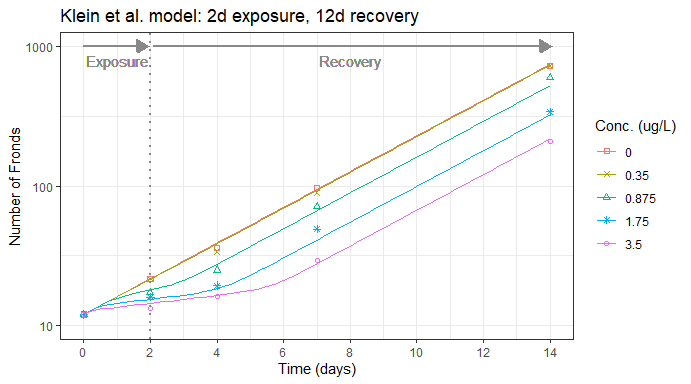Observed model dynamics of the Klein et al. model are identical to published results and fit equally well with the observed frond numbers reported by Hommen et al. A comparison of numerical values of simulated biomass yields:

# simulate using the Schmitt et al. model
df_s <-  simulate_2d(model="schmitt", hmax=0.01)
# simulate using the Klein et al. model
df_k <-  simulate_2d(model="klein", hmax=0.01)

# calculate relative
df_s %>%
mutate(error = 100 * (df_k$BM/BM - 1)) %>% pull(error) %>% abs() %>% max() #>  0.004476714 Numerical biomass values deviate at most by about 0.004% between the two models. It can be concluded that model dynamics and numerical results of both models are identical for the presented scenarios. ### Complex exposure patterns Figure 4 in Schmitt et al. (2013) depicts the growth of Lemna exposed to three exposure patterns of several different concentrations. The patterns include constant exposure, repeating intervals of four days out of seven of exposure, as well as repeating intervals of two days out of seven of exposure. First, the constant exposure pattern (Figure 4a) is simulated: # simulate a specific exposure pattern simulate_pattern <- function(model=c("schmitt","klein"), levels, expo_fun, ...) { model <- match.arg(model) time <- seq(0, 50, 0.2) # output time points # set parameters as needed to replicate Figure 2 from Schmitt et al. if(model == "schmitt") { param$k_phot_fix <- TRUE # unlimited growth
param$k_phot_max <- 0.295 param$EC50 <- 0.39
param$k_resp <- 0 } else if(model == "klein") { param <- metsulfuron$param
param$k_photo_fixed <- TRUE param$k_photo_max <- 0.295
param$EC50_int <- 0.39 param$k_loss <- 0
envir <- metsulfuron$envir } result <- data.frame() for(conc in levels) { exposure <- expo_fun(conc) if(model == "schmitt") { param$Conc <- exposure
out <- calcgrowth(time, c(BM=0.0006, E=1, M_int=0), param=param, hmax=0.01)
out$Area <- out$BM * param$AperBM } else if(model == "klein") { envir$conc <- exposure
out <- lemna(times=time, init=c(BM=0.0006, M_int=0), param=param, envir=envir, hmax=0.01)
out$Area <- out$BM * param$r_A_DW } out$level = as.character(conc)
result <- bind_rows(result, out)
}
result
}

# constant exposure
expo_const <- function(conc=1) {
data.frame(time=c(0, 50), c=c(conc, conc))
}
# exposure concentrations used for constant patterns
levels_const <- c(0, 0.1, 0.25, 0.5, 1)

# simulate constant exposure patterns
df_p1 <- simulate_pattern(model="klein", levels=levels_const, expo_fun=expo_const)

ggplot(df_p1)+
geom_line(aes(time,Area,color=level))+
scale_y_log10(breaks=10^seq(-1,7),minor_breaks=NULL)+
scale_x_continuous(breaks=seq(0,50,10))+
scale_shape_manual(values=c(0,4,2,8,1,3,5))+
coord_cartesian(ylim=c(0.1,10^7))+
theme_bw()+
labs(x="Time (days)", y="Frond area (cm²)", color="Conc. (ug/L)",
title="Klein et al. model: constant exposure")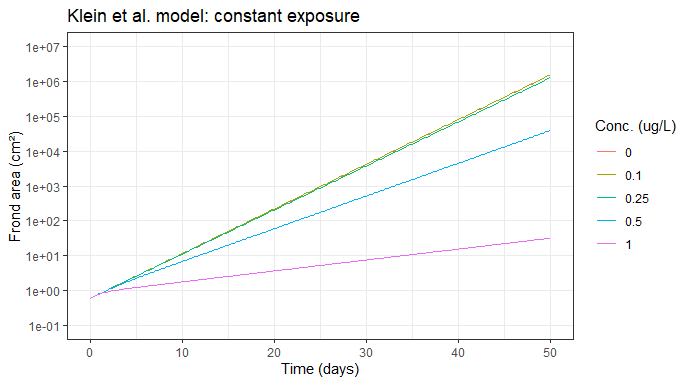Next, the four out of seven days exposure pattern (Figure 4b) is simulated:

# exposure pattern: repeats 4d exposure, 3d recovery for 42 days,
# followed by recovery for 8 days
expo_4of7 <- function(conc=1) {
tim <- c(0,       4, 4.01, 6.99)
exp <- c(conc, conc,    0,    0)

tim_all <- c()
exp_all <- c()
for(i in seq(0,35, 7)) {
tim_all <- c(tim_all, tim+i)
exp_all <- c(exp_all, exp)
}
data.frame("time"=c(tim_all, 50), "conc"=c(exp_all, 0))
}
# exposure concentrations used for 4 out of 7d patterns
levels_4of7 <- c(0, 0.175, 0.438, 0.875, 1.75)

df_p2 <- simulate_pattern(model="klein", levels=levels_4of7, expo_fun=expo_4of7)

ggplot(df_p2)+
geom_line(aes(time,Area,color=level))+
scale_y_log10(breaks=10^seq(-1,7),minor_breaks=NULL)+
scale_x_continuous(breaks=seq(0,50,10))+
scale_shape_manual(values=c(0,4,2,8,1,3,5))+
coord_cartesian(ylim=c(0.1,10^7))+
theme_bw()+
labs(x="Time (days)", y="Frond area (cm²)", color="Conc. (ug/L)",
title="Klein et al. model: 4 out of 7d of exposure")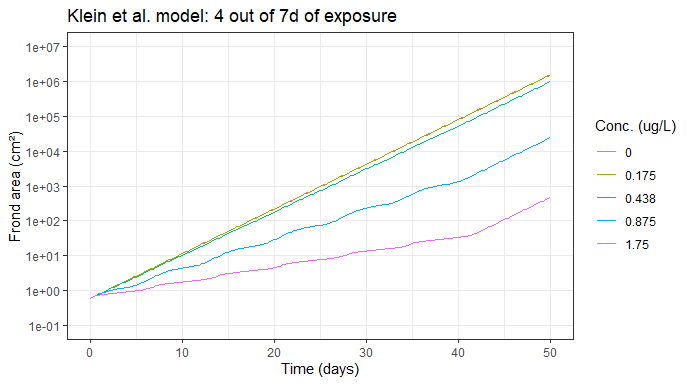Finally, the two out of seven days exposure pattern (Figure 4c) is simulated:

# exposure pattern: repeats 2d exposure, 5d recovery for 42 days,
# followed by recovery for 8 days
expo_2of7 <- function(conc=1) {
tim <- c(0,       2, 2.01, 6.99)
exp <- c(conc, conc,    0,    0)

tim_all <- c()
exp_all <- c()
for(i in seq(0,35, 7)) {
tim_all <- c(tim_all, tim+i)
exp_all <- c(exp_all, exp)
}
data.frame("time"=c(tim_all, 50), "conc"=c(exp_all, 0))
}
# exposure concentrations used for 2 out of 7d patterns
levels_2of7 <- c(0, 0.35, 0.875, 1.75, 3.5)

df_p3 <- simulate_pattern(model="klein", levels=levels_2of7, expo_fun=expo_2of7)

ggplot(df_p3)+
geom_line(aes(time,Area,color=level))+
scale_y_log10(breaks=10^seq(-1,7),minor_breaks=NULL)+
scale_x_continuous(breaks=seq(0,50,10))+
scale_shape_manual(values=c(0,4,2,8,1,3,5))+
coord_cartesian(ylim=c(0.1,10^7))+
theme_bw()+
labs(x="Time (days)", y="Frond area (cm²)", color="Conc. (ug/L)",
title="Klein et al. model: 2 out of 7d of exposure")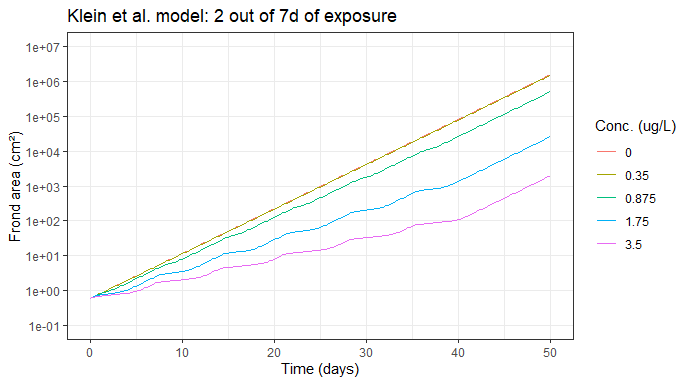Comparing numerical results of the Klein et al. model with the Schmitt et al. implementation yields the following relative errors in percent:

# relative error (%) for the constant exposure pattern
simulate_pattern(model="schmitt", levels=levels_const, expo_fun=expo_const) %>%
mutate(error = 100 * (df_p1$BM/BM - 1)) %>% pull(error) %>% abs() %>% max() #>  0.0005302434 # relative error (%) for the 4 out of 7d exposure pattern simulate_pattern(model="schmitt", levels=levels_4of7, expo_fun=expo_4of7) %>% mutate(error = 100 * (df_p2$BM/BM - 1)) %>%
pull(error) %>%
abs() %>%
max()
#>  0.03496461

# relative error (%) for the 2 out of 7d exposure pattern
simulate_pattern(model="schmitt", levels=levels_2of7, expo_fun=expo_2of7) %>%
mutate(error = 100 * (df_p3$BM/BM - 1)) %>% pull(error) %>% abs() %>% max() #>  0.02197463 It can be observed that the Klein et al. model reproduces the same model dynamics and biomass amounts as reported by Schmitt et al. under unlimited growth conditions. ## Environmental variability scenarios In this section, simulated biomass dynamics of the Klein et al. model are compared to published results under conditions of changing environmental variables. In contrast to the Schmitt et al. model, it applies Liebig’s Law so that growth is controlled by the most limiting resource, i.e. the limiting factor. The environmental variables temperature, global radiation, phosphorus, and nitrate are considered for Liebig’s law (Klein et al. 2015). The following graphs will replicate Figure 5 from Hommen et al. (2015). The simulations make use of exposure time series and time series of environmental variables that were extracted from the three FOCUS Surface Water exposure scenarios D1 Ditch, D2 Ditch, and R3 Stream. Substance specific parameters follow the fitted parameters reported by Schmitt et al. (2013). ### FOCUS D1 Ditch The following code simulates Lemna population growth for the example FOCUS exposure pattern D1 Ditch multiplied by factors from 1 to 100: library(tidyr) # for additional data preparation library(furrr) # for multi-threading capabilities # enable multithreading to reduce processing time future::plan("multisession") rainbow_plot <- function(df, exposure, scale_f, title) { # scale exposure concentration to make it visible in plot df_expo <- exposure %>% mutate(Conc = Conc * scale_f) # do not plot control df_plot <- df %>% filter(factor != "0") # create rainbow plot ggplot(df_plot)+ geom_line(aes(time, BM, color=factor))+ geom_line(aes(Time,Conc), data=df_expo, color="black")+ scale_color_manual(values=setNames(rev(RColorBrewer::brewer.pal(11, "Spectral")), unique(df_plot$factor)))+
scale_y_continuous(breaks=seq(0,200,20))+
scale_x_continuous(breaks=seq(0,390,30))+
coord_cartesian(ylim=c(0,180),expand=FALSE)+
theme_bw()+
labs(x="Time (days)", y="Dry biomass (g dw/m2)", title=title)
}

simulate_focus <- function(scale_f, model, scenario) {
if(model == "schmitt") {
param$k_phot_fix <- FALSE param$mass_per_frond <- 0.0004
param$Conc <- scenario$envir$conc param$Conc$Conc <- param$Conc$Conc * scale_f param$Temp <- scenario$envir$tmp
param$Rad <- scenario$envir$irr res <- calcgrowth(scenario$times, c(BM=scenario$init[["BM"]], E=1, M_int=0), param, hmax=0.01) } else if(model == "klein") { envir <- scenario$envir
envir$conc$Conc <- envir$conc$Conc * scale_f
res <- lemna(scenario, envir=envir, nout=0, hmax=0.01)
}
res$factor <- as.character(scale_f) res } # multiplication factors for the exposure series factors <- c(0, round(10^seq(0,2,0.2), 1)) # simulate using the Schmitt et al. model df_s <- future_map_dfr(factors, simulate_focus, model="schmitt", scenario=focusd1) rainbow_plot(df_s, focusd1$envir$conc, 2000, "D1 Ditch: Schmitt et al. model, L. gibba")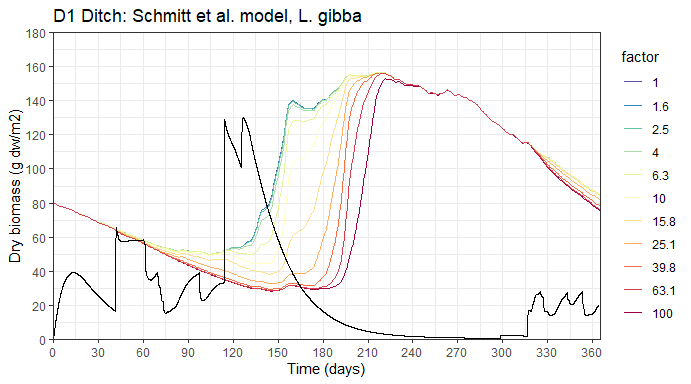# simulate using the Klein et al. model df_k <- future_map_dfr(factors, simulate_focus, model="klein", scenario=focusd1) rainbow_plot(df_k, focusd1$envir$conc, 2000, "D1 Ditch: Klein et al. model, L. gibba")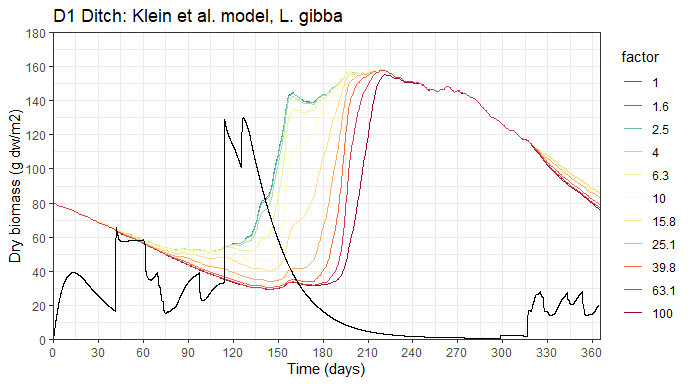It can be observed that the Klein et al. model generates biomass dynamics that are very similar to the Schmitt et al. results. Comparing biomass values for each time point and multiplication factor yields the following graph: bm_dev_plot <- function(res_klein, res_schmitt, title) { fs <- unique(res_klein$factor)

res_schmitt %>%
mutate(error = 100 * (res_klein$BM/BM - 1)) %>% ggplot(aes(time, error, color=factor)) + geom_point() + scale_color_manual(values=setNames(c("black",rev(RColorBrewer::brewer.pal(length(fs) - 1, "Spectral"))), fs)) + scale_x_continuous(breaks=seq(0,390,30)) + theme_bw() + labs(x="Time (days)", y="Relative error (%)", color="Factor", title=paste0(title, ": Deviation of biomass in Klein model relative to Schmitt")) } bm_dev_plot(df_k, df_s, "D1 Ditch")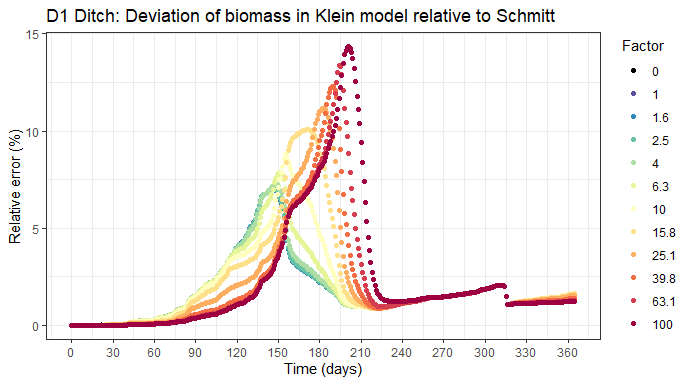For D1 Ditch, the Klein et al. model shows a trend to a slightly larger biomass over the course of the simulated year. Achieved biomass levels are nevertheless very similar in both models. The perceived differences seem to originate from the fact that model results are slightly shifted in time. This observation can be explained by the photosynthesis response function of the Klein et al. model, where growth is controlled by the most limiting factor. Unlike the Schmitt et al. model, where all response functions are multiplied. This allows the population to react quicker to changing environmental conditions and achieve slightly larger biomass in the Klein et al. model. Generally, both models generate similar growth dynamics. The following table reproduces Table 2 from Hommen et al. (2015). It lists the number of days with deviations from controls exceeding different magnitudes (% dev) depending on the exposure multiplication factor in the D1 Ditch scenario: df_k %>% pivot_wider(id_cols=time, names_from=factor, values_from=BM) -> df.wide # table of days with deviations (100*abs(1 - df.wide[-c(1,2)]/df.wide[,c(rep(2,11))])) %>% pivot_longer(1:11, names_to="factor", values_to="dev") %>% group_by(factor) %>% summarize(gt1=sum(dev>1),gt5=sum(dev>5),gt10=sum(dev>10),gt20=sum(dev>20), gt30=sum(dev>30),gt40=sum(dev>40),gt50=sum(dev>50),gt60=sum(dev>60), gt70=sum(dev>70),gt80=sum(dev>80),gt90=sum(dev>90)) %>% arrange(as.numeric(factor)) %>% pivot_longer(cols=2:11, names_to="class", values_to="count") %>% pivot_wider(id_cols=class, names_from=factor, values_from=count) %>% mutate(class = paste0("> ",substring(class, 3))) %>% rename(% dev=class) %>% knitr::kable() % dev 1 1.6 2.5 4 6.3 10 15.8 25.1 39.8 63.1 100 > 1 0 0 15 47 68 131 149 200 209 218 231 > 5 0 0 0 20 45 66 100 126 161 179 187 > 10 0 0 0 0 29 55 75 104 115 130 142 > 20 0 0 0 0 18 35 60 81 98 107 116 > 30 0 0 0 0 0 22 47 68 81 88 98 > 40 0 0 0 0 0 17 38 55 68 76 85 > 50 0 0 0 0 0 0 25 47 56 63 71 > 60 0 0 0 0 0 0 7 34 49 56 62 > 70 0 0 0 0 0 0 0 15 34 43 48 > 80 0 0 0 0 0 0 0 0 0 0 0 Predictions of the Klein et al. model are again very similar to the results reported by Hommen et al. (2015). The number of days with deviations do appear to have a small trend to lower numbers compared to the results of the Schmitt et al. model. As an example: for a multiplication factor of 4, 47 days experience a deviation in biomass of at least 1% compared to 50 days as reported by Hommen et al. ### FOCUS D2 Ditch Simulating Lemna population growth for the example FOCUS exposure pattern D2 Ditch multiplied by factors from 1 to 100: # simulate using the Schmitt et al. model df_s <- future_map_dfr(factors, simulate_focus, model="schmitt", scenario=focusd2) rainbow_plot(df_s, focusd2$envir$conc, 100, "D2 Ditch: Schmitt et al. model, L. gibba")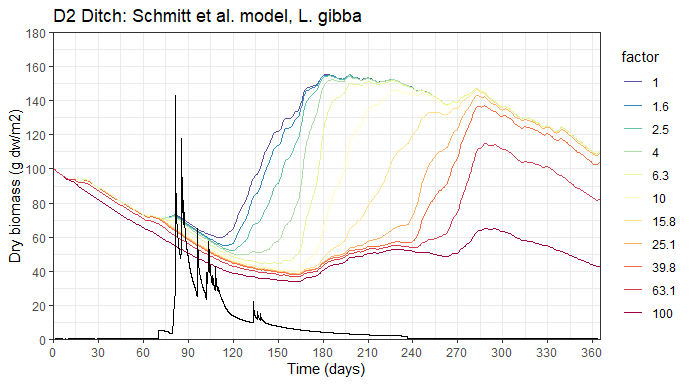# simulate using the Klein et al. model df_k <- future_map_dfr(factors, simulate_focus, model="klein", scenario=focusd2) rainbow_plot(df_k, focusd2$envir$conc, 100, "D2 Ditch: Klein et al. model, L. gibba")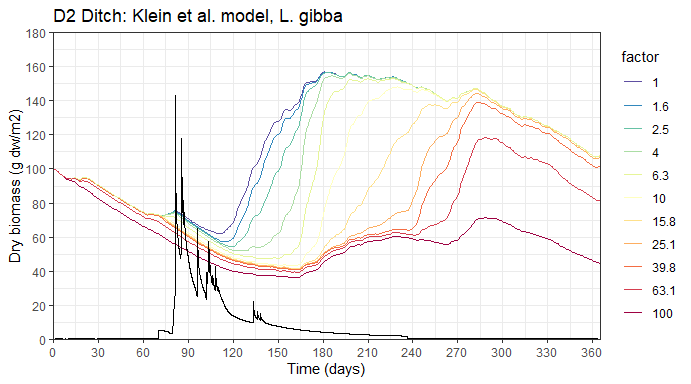bm_dev_plot(df_k, df_s, "D2 Ditch")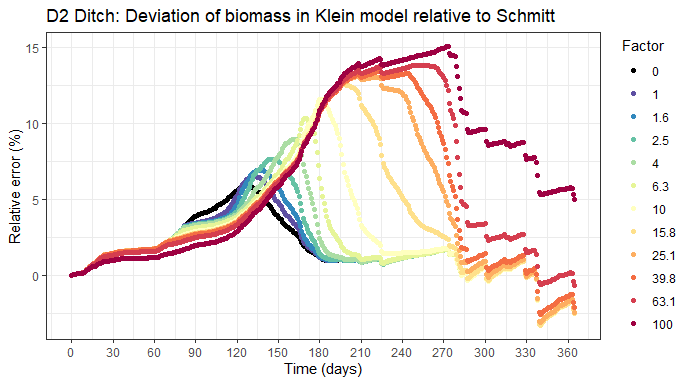Biomass dynamics and absolute numbers are again very similar to the behavior reported by Hommen et al. (2015). Generally, the Klein et al. model shows a trend towards temporary larger biomass values which can be explained by the model reacting quicker to changes in environmental variables. Biomass levels at the end of the simulated year are slightly lower for low and moderate exposure levels than predictions of the Schmitt et al. model. ### FOCUS R3 Stream Simulating Lemna population growth for the example FOCUS exposure pattern R3 Stream multiplied by factors from 1 to 1000: # multiplication factors for the exposure series factors <- c(0, round(10^seq(0,3,0.3), 1)) # simulate using the Schmitt et al. model df_s <- future_map_dfr(factors, simulate_focus, model="schmitt", scenario=focusr3) rainbow_plot(df_s, focusr3$envir$conc, 1000, "R3 Stream: Schmitt et al. model, L. gibba")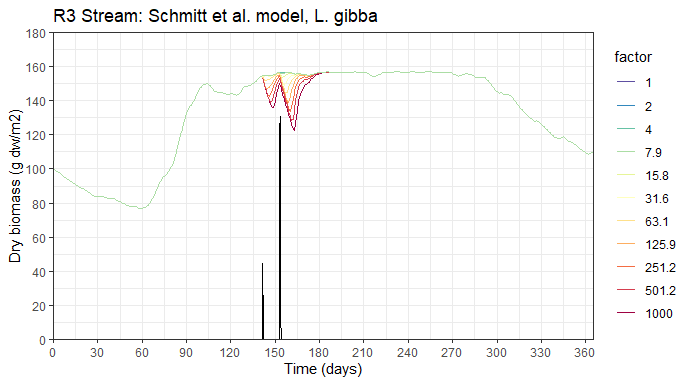# simulate using the Klein et al. model df_k <- future_map_dfr(factors, simulate_focus, model="klein", scenario=focusr3) rainbow_plot(df_k, focusr3$envir\$conc, 1000, "R3 Stream: Klein et al. model, L. gibba")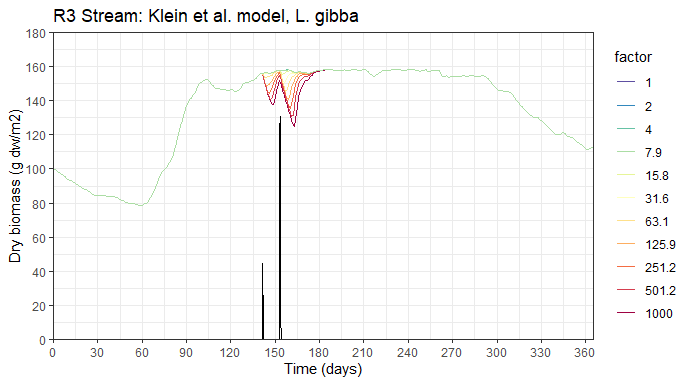bm_dev_plot(df_k, df_s, "R3 Stream")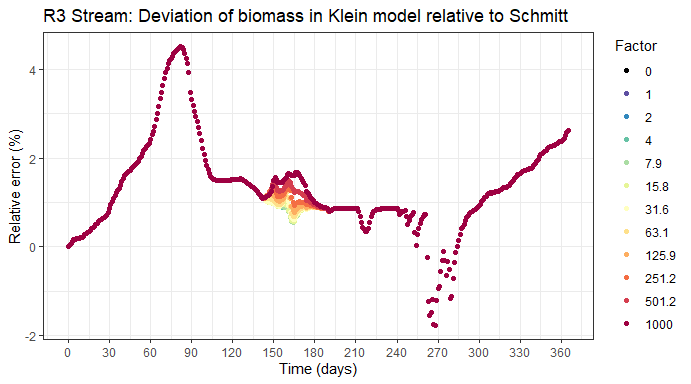# disable multithreading
future::plan("sequential")

Again, biomass dynamics and absolute numbers are very similar to the behavior reported by Hommen et al. (2015) with a small trend towards a larger biomass in the Klein et al. model.

## Compiled code

Model equations implemented as compiled code in C are not part of this verification document for reasons of brevity. However, results of the C code are identical as far as numerical precision allows. This is ensured by automated unit tests which are shipped as part of the lemna source package. The suite of verification tests can be run manually by:

devtools::test(pkg="lemna", filter="verify")

## Acknowledgements

The author would like to thank U. Hommen and S. Heine for providing the original data sets and scripts which were used for past publications, as well as J. Klein and J. Witt for their feedback and suggestions.